# Usage Guide¶

This tutorial covers some basic usage patterns and best-practices to help you get started with Matplotlib.

# sphinx_gallery_thumbnail_number = 3
import matplotlib.pyplot as plt
import numpy as np


## A simple example¶

Matplotlib graphs your data on Figures (i.e., windows, Jupyter widgets, etc.), each of which can contain one or more Axes (i.e., an area where points can be specified in terms of x-y coordinates (or theta-r in a polar plot, or x-y-z in a 3D plot, etc.). The most simple way of creating a figure with an axes is using pyplot.subplots. We can then use Axes.plot to draw some data on the axes:

fig, ax = plt.subplots()  # Create a figure containing a single axes.
ax.plot([1, 2, 3, 4], [1, 4, 2, 3])  # Plot some data on the axes.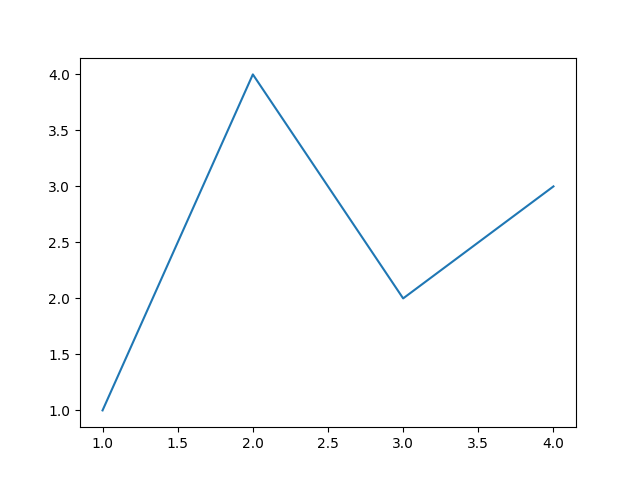Out:

[<matplotlib.lines.Line2D object at 0x7f1559fba2b0>]


Many other plotting libraries or languages do not require you to explicitly create an axes. For example, in MATLAB, one can just do

plot([1, 2, 3, 4], [1, 4, 2, 3])  % MATLAB plot.


and get the desired graph.

In fact, you can do the same in Matplotlib: for each Axes graphing method, there is a corresponding function in the matplotlib.pyplot module that performs that plot on the "current" axes, creating that axes (and its parent figure) if they don't exist yet. So the previous example can be written more shortly as

plt.plot([1, 2, 3, 4], [1, 4, 2, 3])  # Matplotlib plot.Out:

[<matplotlib.lines.Line2D object at 0x7f15297bd9a0>]


## Parts of a Figure¶

Now, let's have a deeper look at the components of a Matplotlib figure.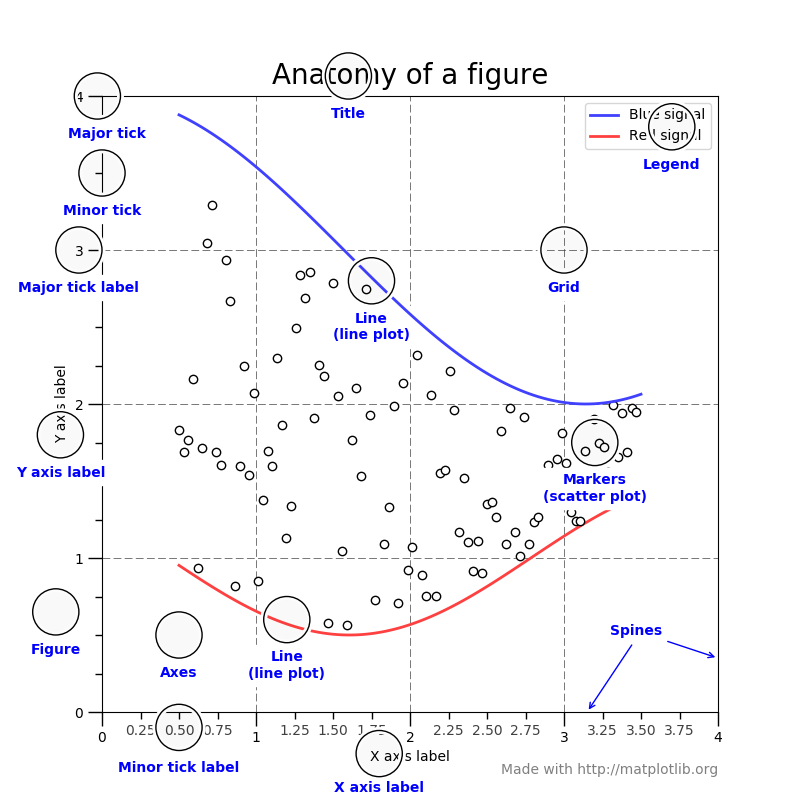### Figure¶

The whole figure. The figure keeps track of all the child Axes, a smattering of 'special' artists (titles, figure legends, etc), and the canvas. (Don't worry too much about the canvas, it is crucial as it is the object that actually does the drawing to get you your plot, but as the user it is more-or-less invisible to you). A figure can contain any number of Axes, but will typically have at least one.

The easiest way to create a new figure is with pyplot:

fig = plt.figure()  # an empty figure with no Axes
fig, ax = plt.subplots()  # a figure with a single Axes
fig, axs = plt.subplots(2, 2)  # a figure with a 2x2 grid of Axes


It's convenient to create the axes together with the figure, but you can also add axes later on, allowing for more complex axes layouts.

### Axes¶

This is what you think of as 'a plot', it is the region of the image with the data space. A given figure can contain many Axes, but a given Axes object can only be in one Figure. The Axes contains two (or three in the case of 3D) Axis objects (be aware of the difference between Axes and Axis) which take care of the data limits (the data limits can also be controlled via the axes.Axes.set_xlim() and axes.Axes.set_ylim() methods). Each Axes has a title (set via set_title()), an x-label (set via set_xlabel()), and a y-label set via set_ylabel()).

The Axes class and its member functions are the primary entry point to working with the OO interface.

### Axis¶

These are the number-line-like objects. They take care of setting the graph limits and generating the ticks (the marks on the axis) and ticklabels (strings labeling the ticks). The location of the ticks is determined by a Locator object and the ticklabel strings are formatted by a Formatter. The combination of the correct Locator and Formatter gives very fine control over the tick locations and labels.

### Artist¶

Basically everything you can see on the figure is an artist (even the Figure, Axes, and Axis objects). This includes Text objects, Line2D objects, collections objects, Patch objects ... (you get the idea). When the figure is rendered, all of the artists are drawn to the canvas. Most Artists are tied to an Axes; such an Artist cannot be shared by multiple Axes, or moved from one to another.

## Types of inputs to plotting functions¶

All of plotting functions expect numpy.array or numpy.ma.masked_array as input. Classes that are 'array-like' such as pandas data objects and numpy.matrix may or may not work as intended. It is best to convert these to numpy.array objects prior to plotting.

For example, to convert a pandas.DataFrame

a = pandas.DataFrame(np.random.rand(4,5), columns = list('abcde'))
a_asarray = a.values


and to convert a numpy.matrix

b = np.matrix([[1, 2], [3, 4]])
b_asarray = np.asarray(b)


## The object-oriented interface and the pyplot interface¶

As noted above, there are essentially two ways to use Matplotlib:

• Explicitly create figures and axes, and call methods on them (the "object-oriented (OO) style").
• Rely on pyplot to automatically create and manage the figures and axes, and use pyplot functions for plotting.

So one can do (OO-style)

x = np.linspace(0, 2, 100)

# Note that even in the OO-style, we use .pyplot.figure to create the figure.
fig, ax = plt.subplots()  # Create a figure and an axes.
ax.plot(x, x, label='linear')  # Plot some data on the axes.
ax.plot(x, x**2, label='quadratic')  # Plot more data on the axes...
ax.plot(x, x**3, label='cubic')  # ... and some more.
ax.set_xlabel('x label')  # Add an x-label to the axes.
ax.set_ylabel('y label')  # Add a y-label to the axes.
ax.set_title("Simple Plot")  # Add a title to the axes.
ax.legend()  # Add a legend.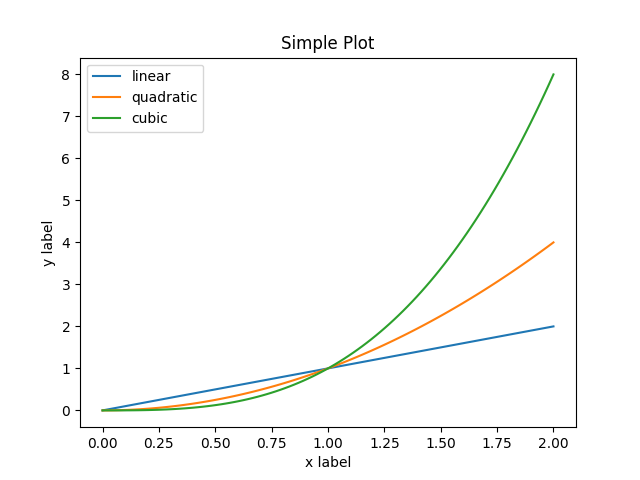Out:

<matplotlib.legend.Legend object at 0x7f152956bc40>


or (pyplot-style)

x = np.linspace(0, 2, 100)

plt.plot(x, x, label='linear')  # Plot some data on the (implicit) axes.
plt.plot(x, x**2, label='quadratic')  # etc.
plt.plot(x, x**3, label='cubic')
plt.xlabel('x label')
plt.ylabel('y label')
plt.title("Simple Plot")
plt.legend()Out:

<matplotlib.legend.Legend object at 0x7f15285e2310>


Actually there is a third approach, for the case where you are embedding Matplotlib in a GUI application, which completely drops pyplot, even for figure creation. We won't discuss it here; see the corresponding section in the gallery for more info (Embedding Matplotlib in graphical user interfaces).

Matplotlib's documentation and examples use both the OO and the pyplot approaches (which are equally powerful), and you should feel free to use either (however, it is preferable pick one of them and stick to it, instead of mixing them). In general, we suggest to restrict pyplot to interactive plotting (e.g., in a Jupyter notebook), and to prefer the OO-style for non-interactive plotting (in functions and scripts that are intended to be reused as part of a larger project).

Note

In older examples, you may find examples that instead used the so-called pylab interface, via from pylab import *. This star-import imports everything both from pyplot and from numpy, so that one could do

x = linspace(0, 2, 100)
plot(x, x, label='linear')
...


for an even more MATLAB-like style. This approach is strongly discouraged nowadays and deprecated; it is only mentioned here because you may still encounter it in the wild.

Typically one finds oneself making the same plots over and over again, but with different data sets, which leads to needing to write specialized functions to do the plotting. The recommended function signature is something like:

def my_plotter(ax, data1, data2, param_dict):
"""
A helper function to make a graph

Parameters
----------
ax : Axes
The axes to draw to

data1 : array
The x data

data2 : array
The y data

param_dict : dict
Dictionary of kwargs to pass to ax.plot

Returns
-------
out : list
list of artists added
"""
out = ax.plot(data1, data2, **param_dict)
return out


which you would then use as:

data1, data2, data3, data4 = np.random.randn(4, 100)
fig, ax = plt.subplots(1, 1)
my_plotter(ax, data1, data2, {'marker': 'x'})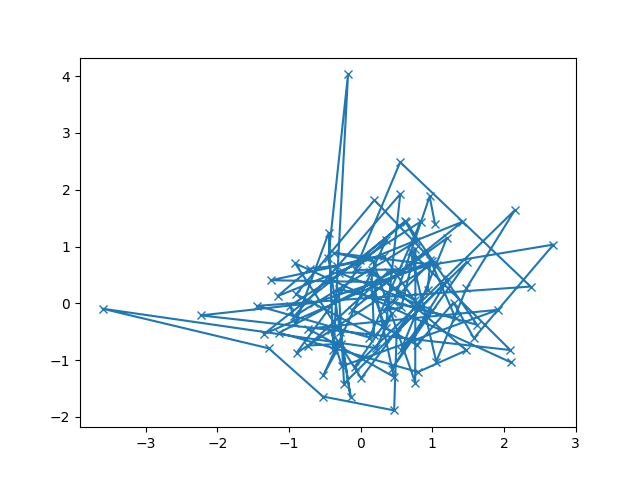Out:

[<matplotlib.lines.Line2D object at 0x7f15285e06d0>]


or if you wanted to have 2 sub-plots:

fig, (ax1, ax2) = plt.subplots(1, 2)
my_plotter(ax1, data1, data2, {'marker': 'x'})
my_plotter(ax2, data3, data4, {'marker': 'o'})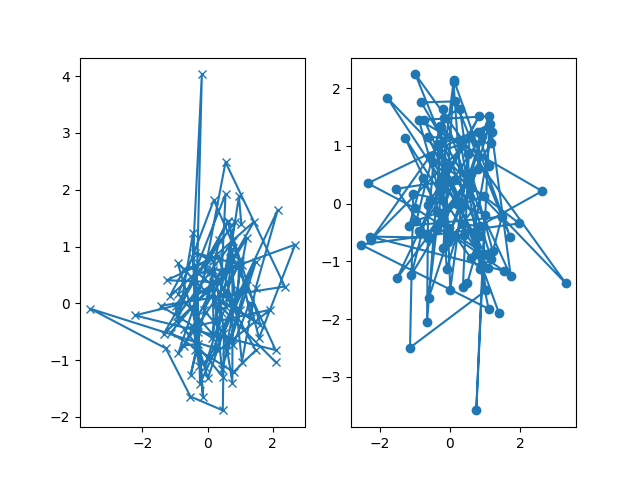Out:

[<matplotlib.lines.Line2D object at 0x7f15296ba580>]


For these simple examples this style seems like overkill, however once the graphs get slightly more complex it pays off.

## Backends¶

### What is a backend?¶

A lot of documentation on the website and in the mailing lists refers to the "backend" and many new users are confused by this term. matplotlib targets many different use cases and output formats. Some people use matplotlib interactively from the python shell and have plotting windows pop up when they type commands. Some people run Jupyter notebooks and draw inline plots for quick data analysis. Others embed matplotlib into graphical user interfaces like wxpython or pygtk to build rich applications. Some people use matplotlib in batch scripts to generate postscript images from numerical simulations, and still others run web application servers to dynamically serve up graphs.

To support all of these use cases, matplotlib can target different outputs, and each of these capabilities is called a backend; the "frontend" is the user facing code, i.e., the plotting code, whereas the "backend" does all the hard work behind-the-scenes to make the figure. There are two types of backends: user interface backends (for use in pygtk, wxpython, tkinter, qt4, or macosx; also referred to as "interactive backends") and hardcopy backends to make image files (PNG, SVG, PDF, PS; also referred to as "non-interactive backends").

### Selecting a backend¶

There are three ways to configure your backend:

1. The rcParams["backend"] (default: 'agg') parameter in your matplotlibrc file
2. The MPLBACKEND environment variable
3. The function matplotlib.use()

A more detailed description is given below.

If multiple of these are configurations are present, the last one from the list takes precedence; e.g. calling matplotlib.use() will override the setting in your matplotlibrc.

If no backend is explicitly set, Matplotlib automatically detects a usable backend based on what is available on your system and on whether a GUI event loop is already running. On Linux, if the environment variable DISPLAY is unset, the "event loop" is identified as "headless", which causes a fallback to a noninteractive backend (agg).

Here is a detailed description of the configuration methods:

1. Setting rcParams["backend"] (default: 'agg') in your matplotlibrc file:

backend : qt5agg   # use pyqt5 with antigrain (agg) rendering

2. Setting the MPLBACKEND environment variable:

You can set the environment variable either for your current shell or for a single script.

On Unix:

> export MPLBACKEND=qt5agg
> python simple_plot.py

> MPLBACKEND=qt5agg python simple_plot.py


On Windows, only the former is possible:

> set MPLBACKEND=qt5agg
> python simple_plot.py


Setting this environment variable will override the backend parameter in any matplotlibrc, even if there is a matplotlibrc in your current working directory. Therefore, setting MPLBACKEND globally, e.g. in your .bashrc or .profile, is discouraged as it might lead to counter-intuitive behavior.

3. If your script depends on a specific backend you can use the function matplotlib.use():

import matplotlib
matplotlib.use('qt5agg')


This should be done before any figure is created; otherwise Matplotlib may fail to switch the backend and raise an ImportError.

Using use will require changes in your code if users want to use a different backend. Therefore, you should avoid explicitly calling use unless absolutely necessary.

### The builtin backends¶

By default, Matplotlib should automatically select a default backend which allows both interactive work and plotting from scripts, with output to the screen and/or to a file, so at least initially you will not need to worry about the backend. The most common exception is if your Python distribution comes without tkinter and you have no other GUI toolkit installed; this happens on certain Linux distributions, where you need to install a Linux package named python-tk (or similar).

If, however, you want to write graphical user interfaces, or a web application server (How to use Matplotlib in a web application server), or need a better understanding of what is going on, read on. To make things a little more customizable for graphical user interfaces, matplotlib separates the concept of the renderer (the thing that actually does the drawing) from the canvas (the place where the drawing goes). The canonical renderer for user interfaces is Agg which uses the Anti-Grain Geometry C++ library to make a raster (pixel) image of the figure; it is used by the Qt5Agg, Qt4Agg, GTK3Agg, wxAgg, TkAgg, and macosx backends. An alternative renderer is based on the Cairo library, used by Qt5Cairo, Qt4Cairo, etc.

For the rendering engines, one can also distinguish between vector or raster renderers. Vector graphics languages issue drawing commands like "draw a line from this point to this point" and hence are scale free, and raster backends generate a pixel representation of the line whose accuracy depends on a DPI setting.

Here is a summary of the matplotlib renderers (there is an eponymous backend for each; these are non-interactive backends, capable of writing to a file):

Renderer Filetypes Description
AGG png raster graphics -- high quality images using the Anti-Grain Geometry engine
PS ps, eps vector graphics -- Postscript output
PDF pdf vector graphics -- Portable Document Format
SVG svg vector graphics -- Scalable Vector Graphics
Cairo png, ps, pdf, svg raster or vector graphics -- using the Cairo library

To save plots using the non-interactive backends, use the matplotlib.pyplot.savefig('filename') method.

To save plots using the non-interactive backends, use the matplotlib.pyplot.savefig('filename') method.

And here are the user interfaces and renderer combinations supported; these are interactive backends, capable of displaying to the screen and of using appropriate renderers from the table above to write to a file:

Backend Description
Qt5Agg Agg rendering in a Qt5 canvas (requires PyQt5). This backend can be activated in IPython with %matplotlib qt5.
ipympl Agg rendering embedded in a Jupyter widget. (requires ipympl). This backend can be enabled in a Jupyter notebook with %matplotlib ipympl.
GTK3Agg Agg rendering to a GTK 3.x canvas (requires PyGObject, and pycairo or cairocffi). This backend can be activated in IPython with %matplotlib gtk3.
macosx Agg rendering into a Cocoa canvas in OSX. This backend can be activated in IPython with %matplotlib osx.
TkAgg Agg rendering to a Tk canvas (requires TkInter). This backend can be activated in IPython with %matplotlib tk.
nbAgg Embed an interactive figure in a Jupyter classic notebook. This backend can be enabled in Jupyter notebooks via %matplotlib notebook.
WebAgg On show() will start a tornado server with an interactive figure.
GTK3Cairo Cairo rendering to a GTK 3.x canvas (requires PyGObject, and pycairo or cairocffi).
Qt4Agg Agg rendering to a Qt4 canvas (requires PyQt4 or pyside). This backend can be activated in IPython with %matplotlib qt4.
wxAgg Agg rendering to a wxWidgets canvas (requires wxPython 4). This backend can be activated in IPython with %matplotlib wx.

Note

The names of builtin backends case-insensitive; e.g., 'Qt5Agg' and 'qt5agg' are equivalent.

#### ipympl¶

The Jupyter widget ecosystem is moving too fast to support directly in Matplotlib. To install ipympl

pip install ipympl
jupyter nbextension enable --py --sys-prefix ipympl


or

conda install ipympl -c conda-forge


See jupyter-matplotlib for more details.

#### GTK and Cairo¶

GTK3 backends (both GTK3Agg and GTK3Cairo) depend on Cairo (pycairo>=1.11.0 or cairocffi).

#### How do I select PyQt4 or PySide?¶

The QT_API environment variable can be set to either pyqt or pyside to use PyQt4 or PySide, respectively.

Since the default value for the bindings to be used is PyQt4, Matplotlib first tries to import it, if the import fails, it tries to import PySide.

### Using non-builtin backends¶

More generally, any importable backend can be selected by using any of the methods above. If name.of.the.backend is the module containing the backend, use module://name.of.the.backend as the backend name, e.g. matplotlib.use('module://name.of.the.backend').

## What is interactive mode?¶

Use of an interactive backend (see What is a backend?) permits--but does not by itself require or ensure--plotting to the screen. Whether and when plotting to the screen occurs, and whether a script or shell session continues after a plot is drawn on the screen, depends on the functions and methods that are called, and on a state variable that determines whether matplotlib is in "interactive mode". The default Boolean value is set by the matplotlibrc file, and may be customized like any other configuration parameter (see Customizing Matplotlib with style sheets and rcParams). It may also be set via matplotlib.interactive(), and its value may be queried via matplotlib.is_interactive(). Turning interactive mode on and off in the middle of a stream of plotting commands, whether in a script or in a shell, is rarely needed and potentially confusing, so in the following we will assume all plotting is done with interactive mode either on or off.

Note

Major changes related to interactivity, and in particular the role and behavior of show(), were made in the transition to matplotlib version 1.0, and bugs were fixed in 1.0.1. Here we describe the version 1.0.1 behavior for the primary interactive backends, with the partial exception of macosx.

Interactive mode may also be turned on via matplotlib.pyplot.ion(), and turned off via matplotlib.pyplot.ioff().

Note

Interactive mode works with suitable backends in ipython and in the ordinary python shell, but it does not work in the IDLE IDE. If the default backend does not support interactivity, an interactive backend can be explicitly activated using any of the methods discussed in What is a backend?.

### Interactive example¶

From an ordinary python prompt, or after invoking ipython with no options, try this:

import matplotlib.pyplot as plt
plt.ion()
plt.plot([1.6, 2.7])


This will pop up a plot window. Your terminal prompt will remain active, so that you can type additional commands such as:

plt.title("interactive test")
plt.xlabel("index")


On most interactive backends, the figure window will also be updated if you change it via the object-oriented interface. E.g. get a reference to the Axes instance, and call a method of that instance:

ax = plt.gca()
ax.plot([3.1, 2.2])


If you are using certain backends (like macosx), or an older version of matplotlib, you may not see the new line added to the plot immediately. In this case, you need to explicitly call draw() in order to update the plot:

plt.draw()


### Non-interactive example¶

Start a fresh session as in the previous example, but now turn interactive mode off:

import matplotlib.pyplot as plt
plt.ioff()
plt.plot([1.6, 2.7])


Nothing happened--or at least nothing has shown up on the screen (unless you are using macosx backend, which is anomalous). To make the plot appear, you need to do this:

plt.show()


Now you see the plot, but your terminal command line is unresponsive; the pyplot.show() command blocks the input of additional commands until you manually kill the plot window.

What good is this--being forced to use a blocking function? Suppose you need a script that plots the contents of a file to the screen. You want to look at that plot, and then end the script. Without some blocking command such as show(), the script would flash up the plot and then end immediately, leaving nothing on the screen.

In addition, non-interactive mode delays all drawing until show() is called; this is more efficient than redrawing the plot each time a line in the script adds a new feature.

Prior to version 1.0, show() generally could not be called more than once in a single script (although sometimes one could get away with it); for version 1.0.1 and above, this restriction is lifted, so one can write a script like this:

import numpy as np
import matplotlib.pyplot as plt

plt.ioff()
for i in range(3):
plt.plot(np.random.rand(10))
plt.show()


which makes three plots, one at a time. I.e. the second plot will show up, once the first plot is closed.

### Summary¶

In interactive mode, pyplot functions automatically draw to the screen.

When plotting interactively, if using object method calls in addition to pyplot functions, then call draw() whenever you want to refresh the plot.

Use non-interactive mode in scripts in which you want to generate one or more figures and display them before ending or generating a new set of figures. In that case, use show() to display the figure(s) and to block execution until you have manually destroyed them.

## Performance¶

Whether exploring data in interactive mode or programmatically saving lots of plots, rendering performance can be a painful bottleneck in your pipeline. Matplotlib provides a couple ways to greatly reduce rendering time at the cost of a slight change (to a settable tolerance) in your plot's appearance. The methods available to reduce rendering time depend on the type of plot that is being created.

### Line segment simplification¶

For plots that have line segments (e.g. typical line plots, outlines of polygons, etc.), rendering performance can be controlled by the path.simplify and path.simplify_threshold parameters in your matplotlibrc file (see Customizing Matplotlib with style sheets and rcParams for more information about the matplotlibrc file). The path.simplify parameter is a boolean indicating whether or not line segments are simplified at all. The path.simplify_threshold parameter controls how much line segments are simplified; higher thresholds result in quicker rendering.

The following script will first display the data without any simplification, and then display the same data with simplification. Try interacting with both of them:

import numpy as np
import matplotlib.pyplot as plt
import matplotlib as mpl

# Setup, and create the data to plot
y = np.random.rand(100000)
y[50000:] *= 2
y[np.logspace(1, np.log10(50000), 400).astype(int)] = -1
mpl.rcParams['path.simplify'] = True

mpl.rcParams['path.simplify_threshold'] = 0.0
plt.plot(y)
plt.show()

mpl.rcParams['path.simplify_threshold'] = 1.0
plt.plot(y)
plt.show()


Matplotlib currently defaults to a conservative simplification threshold of 1/9. If you want to change your default settings to use a different value, you can change your matplotlibrc file. Alternatively, you could create a new style for interactive plotting (with maximal simplification) and another style for publication quality plotting (with minimal simplification) and activate them as necessary. See Customizing Matplotlib with style sheets and rcParams for instructions on how to perform these actions.

The simplification works by iteratively merging line segments into a single vector until the next line segment's perpendicular distance to the vector (measured in display-coordinate space) is greater than the path.simplify_threshold parameter.

Note

Changes related to how line segments are simplified were made in version 2.1. Rendering time will still be improved by these parameters prior to 2.1, but rendering time for some kinds of data will be vastly improved in versions 2.1 and greater.

### Marker simplification¶

Markers can also be simplified, albeit less robustly than line segments. Marker simplification is only available to Line2D objects (through the markevery property). Wherever Line2D construction parameters are passed through, such as matplotlib.pyplot.plot() and matplotlib.axes.Axes.plot(), the markevery parameter can be used:

plt.plot(x, y, markevery=10)


The markevery argument allows for naive subsampling, or an attempt at evenly spaced (along the x axis) sampling. See the Markevery Demo for more information.

### Splitting lines into smaller chunks¶

If you are using the Agg backend (see What is a backend?), then you can make use of the agg.path.chunksize rc parameter. This allows you to specify a chunk size, and any lines with greater than that many vertices will be split into multiple lines, each of which has no more than agg.path.chunksize many vertices. (Unless agg.path.chunksize is zero, in which case there is no chunking.) For some kind of data, chunking the line up into reasonable sizes can greatly decrease rendering time.

The following script will first display the data without any chunk size restriction, and then display the same data with a chunk size of 10,000. The difference can best be seen when the figures are large, try maximizing the GUI and then interacting with them:

import numpy as np
import matplotlib.pyplot as plt
import matplotlib as mpl
mpl.rcParams['path.simplify_threshold'] = 1.0

# Setup, and create the data to plot
y = np.random.rand(100000)
y[50000:] *= 2
y[np.logspace(1, np.log10(50000), 400).astype(int)] = -1
mpl.rcParams['path.simplify'] = True

mpl.rcParams['agg.path.chunksize'] = 0
plt.plot(y)
plt.show()

mpl.rcParams['agg.path.chunksize'] = 10000
plt.plot(y)
plt.show()


### Legends¶

The default legend behavior for axes attempts to find the location that covers the fewest data points (loc='best'). This can be a very expensive computation if there are lots of data points. In this case, you may want to provide a specific location.

### Using the fast style¶

The fast style can be used to automatically set simplification and chunking parameters to reasonable settings to speed up plotting large amounts of data. It can be used simply by running:

import matplotlib.style as mplstyle
mplstyle.use('fast')


It is very light weight, so it plays nicely with other styles, just make sure the fast style is applied last so that other styles do not overwrite the settings:

mplstyle.use(['dark_background', 'ggplot', 'fast'])


Keywords: matplotlib code example, codex, python plot, pyplot Gallery generated by Sphinx-Gallery